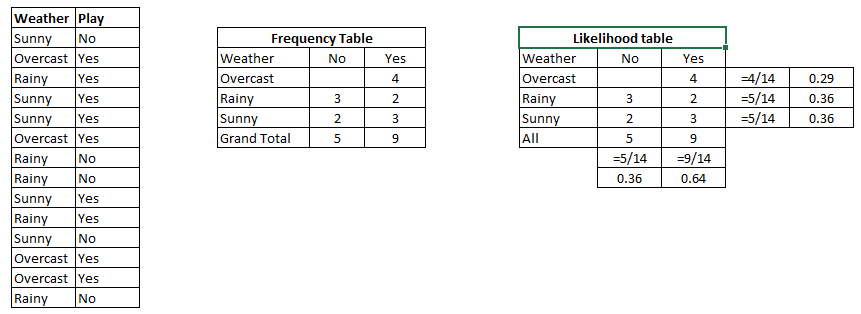## [机器学习]-朴素贝叶斯-最简单的入门实战例子 转

o
osc_w9s1w4o0

1.朴素贝叶斯是如何工作的？

2.朴素贝叶斯的理论基础是什么？

3.朴素贝叶斯的优缺点是什么？

4.一个实战例子

5.使用朴素贝叶斯模型的一些建议P(Yes | Sunny) = P( Sunny | Yes) * P(Yes) / P (Sunny)    （什么？为什么这样计算，下面会介绍^_^）

P (Sunny |Yes) = 3/9 = 0.33, P(Sunny) = 5/14 = 0.36, P( Yes)= 9/14 = 0.64

P(X,Y) = P(X)P(Y)

P(Y|X) = P(X,Y)/P(X)

P(Y|X) = P(X|Y)P(Y)/P(X)

``P(C|F1F2...Fn) = P(F1F2...Fn|C)P(C) / P(F1F2...Fn)``

``P(F1F2...Fn|C)P(C)``

``P(F1F2...Fn|C)P(C) = P(F1|C)P(F2|C) ... P(Fn|C)P(C)``

• 简单、运算量小、在拥有大量分类的数据集上仍然表现很好
• 当数据的各个属性互相独立的假设成立，朴素贝叶斯比逻辑回归等模型表现更好，并且朴素贝叶斯需要更少的训练数据
• 与数值变量相比，朴素贝叶斯在非数值变量的训练集上表现更好，因为对于数值型变量，一般假设数据符合正太分布（这个假设是一个很强的假设，大多数数据集并不相符）

• 如果一个变量的某个值在测试集中有，在训练集中没有，计算结果将是0概率，无法做出分类，为了解决这个问题，需要引入平滑处理，比较简单的是拉普拉斯平滑
• 朴素贝叶斯假定每个变量之间是相互独立的，但是现实中的数据往往都具有一定的相关性，很少有完全独立的

``````import numpy as np

def getDataSet():
"""
加载训练数据, postingList是所有的训练集, 每一个列表代表一条言论, 一共有8条言论 classVec代表每一条言论的类别,
0是正常, 1是有侮辱性 返回 言论和类别
:return:
"""
postingList=[['my', 'dog', 'has', 'flea', 'problems', 'help', 'please'],
['maybe', 'not', 'take', 'him', 'to', 'dog', 'park', 'stupid'],
['my', 'dalmation', 'is', 'so', 'cute', 'I', 'love', 'him'],
['stop', 'posting', 'stupid', 'worthless', 'garbage'],
['mr', 'licks', 'ate', 'my', 'steak', 'how', 'to', 'stop', 'him'],
['quit', 'buying', 'worthless', 'dog', 'food', 'stupid']]
labels = [0,1,0,1,0,1]
return postingList,labels

def createVocabList(dataSet):
"""
创建词汇表, 就是把这个文档中所有的单词不重复的放在一个列表里面
:param dataSet:
:return:
"""
vocabSet = set([])
for data in dataSet:
vocabSet = vocabSet | set(data)
return list(vocabSet)

def vectorize(vocabSet,dataSet):
"""
制作词向量矩阵
将每一个文档转换为词向量, 然后放入矩阵中
:param vocabSet:
:param dataSet:
:return:
"""
vocab =  * len(vocabSet)
for data in dataSet:
vocab[vocabSet.index(data)] = 1
return vocab

def trainN(X_train,y_train):
"""
制作贝叶斯分类器
:param X_train:
:param y_train:
:return:
"""
num = len(X_train)   #有多少记录
numvocab = len(X_train) #词向量的大小
p0Num = np.ones(numvocab) #统计非侮辱类的相关单词频数 加入了拉普拉斯平滑
p1Num = np.ones(numvocab) #统计侮辱类的相关单词频数
p0Sum = 2
p1Sum = 2
pA = sum(y_train) / num                   #先验概率
for i in range(num):
if y_train[i]==0:   #统计属于非侮辱类的条件概率所需的数据
# p0Sum += sum(X_train[i])
p0Sum += 1
p0Num += X_train[i]
else:               #统计属于侮辱类的条件概率所需的数据
# p1Sum += sum(X_train[i])
p1Sum += 1
p1Num += X_train[i]

# 为了防止下溢出，计算条件概率的对数
p0 = np.log(p0Num / p0Sum)      #频数除以总数 得到概率
p1 = np.log(p1Num / p1Sum)
return p0,p1,pA

def classify(testMat,p0,p1,pA):
"""
进行分类
:param testMat:
:param p0:
:param p1:
:param pA:
:return:
"""
p0Score = sum(testMat * p0) + np.log(pA)
p1Score = sum(testMat * p1) + np.log(1-pA)
if p0Score > p1Score:
return 0
else:
return 1

if __name__=='__main__':
dataSet,label = getDataSet()
vocabSet = createVocabList(dataSet)
trainMat = []
for elem in dataSet:
trainMat.append(vectorize(vocabSet,elem))
# print(trainMat)
p0,p1,pA = trainN(trainMat,label)
test1= ['love', 'my', 'dalmation']
test2= ['stupid', 'garbage','love']
test1_vocab = np.array(vectorize(vocabSet,test1))
test2_vocab = np.array(vectorize(vocabSet,test2))
result1 = classify(test1_vocab,p0,p1,pA)
result2 = classify(test2_vocab,p0,p1,pA)
if result1==1:
print(test1,"属于：侮辱类")
else:
print(test1, "属于：非侮辱类")
print("------------------------------------------")
if result2==1:
print(test2,"属于：侮辱类")
else:
print(test2, "属于：非侮辱类")``````

结果：

``````['love', 'my', 'dalmation'] 属于：非侮辱类
------------------------------------------
['stupid', 'garbage', 'love'] 属于：侮辱类``````

• 如果连续型变量不符合正态分布，需要使用一些方法转换为正太分布
• 删除相关属性，如果相关属性多次参与计算概率，会导致该属性出现的概率变大
• 如果测试数据出现某个变量值在训练集为0时，记得使用拉普拉斯平滑

o

### osc_w9s1w4o0#### 暂无文章

Java线程池

nullpointerxyz
29分钟前
35
0
Python笔记：用Python制作二维码

tengyulong
41分钟前
0
0
Redis-初体验/数据结构

44分钟前
15
0

fyin1314

29
0

19
0